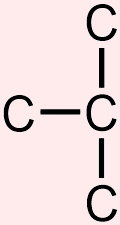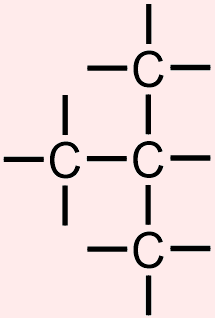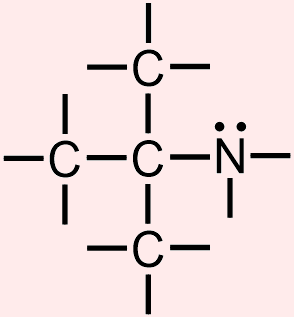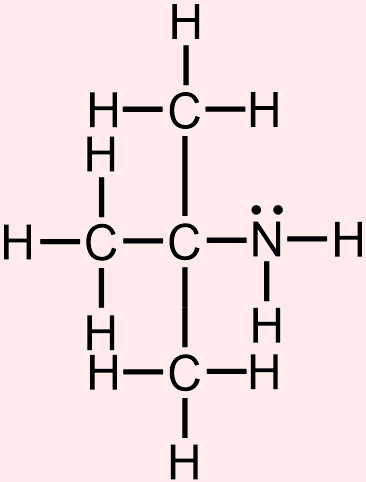# 10.7: Organic Structural Representations: Additional Examples

$$\newcommand{\vecs}{\overset { \rightharpoonup} {\mathbf{#1}} }$$ $$\newcommand{\vecd}{\overset{-\!-\!\rightharpoonup}{\vphantom{a}\smash {#1}}}$$$$\newcommand{\id}{\mathrm{id}}$$ $$\newcommand{\Span}{\mathrm{span}}$$ $$\newcommand{\kernel}{\mathrm{null}\,}$$ $$\newcommand{\range}{\mathrm{range}\,}$$ $$\newcommand{\RealPart}{\mathrm{Re}}$$ $$\newcommand{\ImaginaryPart}{\mathrm{Im}}$$ $$\newcommand{\Argument}{\mathrm{Arg}}$$ $$\newcommand{\norm}{\| #1 \|}$$ $$\newcommand{\inner}{\langle #1, #2 \rangle}$$ $$\newcommand{\Span}{\mathrm{span}}$$ $$\newcommand{\id}{\mathrm{id}}$$ $$\newcommand{\Span}{\mathrm{span}}$$ $$\newcommand{\kernel}{\mathrm{null}\,}$$ $$\newcommand{\range}{\mathrm{range}\,}$$ $$\newcommand{\RealPart}{\mathrm{Re}}$$ $$\newcommand{\ImaginaryPart}{\mathrm{Im}}$$ $$\newcommand{\Argument}{\mathrm{Arg}}$$ $$\newcommand{\norm}{\| #1 \|}$$ $$\newcommand{\inner}{\langle #1, #2 \rangle}$$ $$\newcommand{\Span}{\mathrm{span}}$$$$\newcommand{\AA}{\unicode[.8,0]{x212B}}$$

Learning Objectives
• Identify the type of structural notation in which an organic molecule is represented.
• Represent a given organic image using a different type of structural notation.
Exercise $$\PageIndex{1}$$

Consider the following organic structural representation.1. Identify the type of notation in which the given structure is drawn.
2. Write the molecular formula of the organic molecule that is represented by this picture.
3. Represent the molecular image that is shown above using bond-line notation.
The given structure, which explicitly shows all of the stick bonds that are located between consecutive pairs of elemental symbols, reflects the two-dimensional connectivity of the atoms that are present in the corresponding organic molecule and, therefore, is drawn using expanded structural notation.
A molecular formula is an abbreviation that summarizes both the identities and quantities of elements that are present in an organic chemical.  In order to determine this information for a molecule that is represented using expanded structural notation, the elemental symbols that are shown, which indicate the types of elements that are found in the corresponding substance, can be counted.  Since the elemental symbol "C" is written five times in the given structure, and "H" appears ten times, the corresponding molecule contains five carbon atoms and ten hydrogen atoms.

Because an organic molecule must be comprised of carbon, C, and usually contains hydrogen, H, these elemental symbols, as well as their corresponding subscripts, are written first and second, respectively, when developing a molecular formula.  Therefore, C5H10 is the chemically-correct molecular formula of the organic substance that is represented by the given expanded structure.  These subscripts should not be divided by 5, even though it is mathematically-possible to do so, as the resultant formula, CH2, would not be consistent with the image that is shown above.
Bond-line structures, which use "zig-zag" lines to represent the bonds that exist between consecutive carbon atoms, are generated by eliminating
• all lone pairs,
• all non-metal/hydrogen bonds,
• all elemental symbols that represent carbon, C, and
• any hydrogen, H, elemental symbols that are associated with carbon atoms
from an image that is drawn using a more detailed notation.  After removing all of these structural components, which are indicated by light blue "" symbols, from the expanded structure that is reproduced below,the following bonds remain.In a bond-line structure, these remaining single bonds are represented using "zig-zag" lines that are analogous to those that are used in VSEPR notation.  Furthermore, because the carbon atoms that are present in the given structure are arranged in a cyclic shape, the lines that are incorporated into the bond-line structure that is being developed must be drawn in the same orientation, in order to accurately reflect the connectivity of the corresponding molecule.  The image that is shown below is the chemically-correct bond-line representation of the given expanded structure.Exercise $$\PageIndex{2}$$

Consider the following organic structural representation.1. Identify the type of notation in which the given structure is drawn.
2. Write the molecular formula of the organic molecule that is represented by this picture.
3. Represent the molecular image that is shown above using condensed structural notation.
The given structure, which explicitly shows all of the stick bonds that are located between consecutive pairs of elemental symbols, reflects the two-dimensional connectivity of the atoms that are present in the corresponding organic molecule and, therefore, is drawn using expanded structural notation.
A molecular formula is an abbreviation that summarizes both the identities and quantities of elements that are present in an organic chemical.  In order to determine this information for a molecule that is represented using expanded structural notation, the elemental symbols that are shown, which indicate the types of elements that are found in the corresponding substance, can be counted.  Since the elemental symbol "C" is written 7 times in the given structure, and "H" appears 14 times, the corresponding molecule contains 7 carbon atoms and 14 hydrogen atoms.

Because an organic molecule must be comprised of carbon, C, and usually contains hydrogen, H, these elemental symbols, as well as their corresponding subscripts, are written first and second, respectively, when developing a molecular formula.  Therefore, C7H14 is the chemically-correct molecular formula of the organic substance that is represented by the given expanded structure.  These subscripts should not be divided by 7, even though it is mathematically-possible to do so, as the resultant formula, CH2, would not be consistent with the image that is shown above.
Condensed structures, which use a "formula-style" format to indicate the number of hydrogens that are bonded to a particular non-metal, are generated by eliminating
• all lone pairs,
• all carbon/hydrogen bonds, and
• all single bonds that are written in a horizontal, or left-to-right, direction
from an image that is drawn using a more detailed notation.  After removing all of these structural components, which are indicated by light blue "" symbols, from the expanded structure that is reproduced below,the following elemental symbols and bonds remain.  The locations and relative orientations of the double bond, as well as the remaining vertical single bond, are maintained and, therefore, are directly translated from the given picture to the corresponding positions in the condensed structure that is being developed, as shown below.Finally, all hydrogen groupings are represented using a "JHQ" format, in which the generic placeholder letter "J" is replaced with the elemental symbol of a non-metal, and the "Q" subscript corresponds to the number of hydrogens that are bonded to that atom.  The five carbon atoms that are represented in the main "chain" of the given structure are bonded to three, two, one, one, and two hydrogens, respectively.  Because values of "1" are usually implicitly-understood in chemistry, these groupings are condensed as "CH3," "CH2," "CH," "CH," and "CH2" in the structure that is being developed.  Furthermore, since the carbon atoms that are located in the bottom "branch" of the provided structure are associated with two and three hydrogen atoms, respectively, these portions of the corresponding organic molecule are represented as "CH2" and "CH3" units, as shown in the following chemically-correct condensed representation of the given expanded structure.Exercise $$\PageIndex{3}$$

Consider the following organic structural representation.1. Identify the type of notation in which the given structure is drawn.
2. Write the molecular formula of the organic molecule that is represented by this picture.
3. Represent the molecular image that is shown above using expanded structural notation.
The given structure, which uses a "formula-style" format to indicate the number of hydrogens that are bonded to a particular non-metal, only explicitly shows the vertical single bonds that are present between consecutive pairs of elemental symbols and, therefore, is drawn using condensed structural notation.
A molecular formula is an abbreviation that summarizes both the identities and quantities of elements that are present in an organic chemical.  In order to determine this information for a molecule that is represented using condensed structural notation, the subscripts that are shown on the elemental symbols, which indicate the types of elements that are found in the corresponding substance, can be added.  Since the elemental symbol "C" is written 4 times in the given structure, "N" appears once, and subscripts of 3, 3, 3, and 2 are associated with the different hydrogen, H, groupings, the corresponding molecule contains 4 carbon atoms, 1 nitrogen, and 11 hydrogen atoms.

Because an organic molecule must be comprised of carbon, C, and usually contains hydrogen, H, these elemental symbols, as well as their corresponding subscripts, are written first and second, respectively, when developing a molecular formula.  Since nitrogen, N, is classified as a heteroatom, its elemental symbol is written last.  Therefore, because values of "1" are usually implicitly-understood in chemistry, C4H11N is the chemically-correct molecular formula of the organic substance that is represented by the given condensed structure.
An expanded structure reflects the two-dimensional connectivity of an organic molecule by explicitly showing the symbols of all of the atoms that are contained in that substance, as well as all of the stick bonds that are located between, and the lone pairs that are associated with, each of those elemental symbols.

Because all organic molecules must contain carbon, C, this element is written first when symbolizing an organic substance using expanded structural notation.  The carbon atoms that are present in the given image are arranged in a sideways "T"-shape.  Therefore, in order to accurately reflect the connectivity of the corresponding molecule, these elemental symbols are incorporated into the expanded structure that is being developed in the same relative positions, and consecutive pairs of symbols are connected to one another using two-dimensional stick bonds, as shown below.Recall that carbon forms four bonds when present in an organic molecule.  Therefore, in order to achieve this preferred bonding configuration for each carbon atom that is represented in the structure that is being developed, one more stick bond is added to the central "C," and three additional lines are drawn on the remaining elemental symbols, and as shown below.The elemental symbol of nitrogen, N, is then incorporated into the structure that is being developed.  Because this heteroatom is bonded to the central carbon in the molecule that is represented by the given structure, the elemental symbol "N" is written in the unoccupied position at the end of the bond that is associated with this carbon atom in the structure that is shown above.  Additionally, recall that an atom of nitrogen, N, contains three unpaired valence electrons and one set of paired electrons and, consequently, forms three covalent bonds and bears one lone pair when present in an organic molecule.  Therefore, in order to achieve this preferred bonding configuration, one lone pair and two more lines are drawn on the elemental symbol of nitrogen, N, that is present in the structure that is being developed, as shown below.Finally, in order to generate the following chemically-correct expanded structural representation of the given condensed image, the elemental symbol of hydrogen, H, is written in all of the unoccupied positions at the ends of the bonds that have been drawn.Exercise $$\PageIndex{4}$$

Consider the following organic structural representation.1. Identify the type of notation in which the given structure is drawn.
2. Write the molecular formula of the organic molecule that is represented by this picture.
3. Represent the molecular image that is shown above using VSEPR notation.
The given structure, which uses "zig-zag" lines to represent the bonds that exist between consecutive carbon atoms, does not explicitly show the elemental symbols of carbon or hydrogen and, therefore, is drawn using bond-line notation.
A molecular formula is an abbreviation that summarizes both the identities and quantities of elements that are present in an organic chemical.  In bond-line notation, a carbon atom is understood to be located at every point at which two or more bonds meet and on the end of every line that is not explicitly-associated with a heteroatom.  Therefore, since the given structure has two "ends" and four internal places at which consecutive bonds connect with one another, the molecule that is represented above contains a total of six carbon atoms.  Furthermore, each of these carbons is assumed to be bonded to enough hydrogens to achieve its preferred four-bond configuration.  As one bond is explicitly-drawn at each of the "ends" of the given image, three bonds to hydrogen are implicitly-understood to be associated with each of these terminating points.  Additionally, because each internal carbon that is represented in this bond-line structure is shown to be bonded to two additional atoms, two hydrogens are also present on each of these carbons.  Therefore, a total of 14 hydrogens are present in the organic molecule that is symbolized by the image that is shown above.

Because an organic molecule must be comprised of carbon, C, and usually contains hydrogen, H, these elemental symbols, as well as their corresponding subscripts, are written first and second, respectively, when developing a molecular formula.  Therefore, C6H14 is the chemically-correct molecular formula of the organic substance that is represented by the given bond-line structure.  These subscripts should not be divided by 2, even though it is mathematically-possible to do so, as the resultant formula, C3H7, would not be consistent with the image that is shown above.
A VSEPR structure reflects the three-dimensional spatial orientation of an organic molecule and its constituent atoms by explicitly showing the symbols of all of the elements that are contained in that substance, as well as all of the stick, wedged, and dashed bonds that are located between those elemental symbols.

Because all organic molecules must contain carbon, C, this element is written first when symbolizing an organic substance using VSEPR notation.  The carbon atoms that are present in the given image are arranged in a linear "chain."  Therefore, in order to accurately reflect the connectivity of the corresponding molecule, these elemental symbols, which, as stated above, are explicitly-written in a VSEPR picture, are incorporated into the structure that is being developed in the same relative positions.  Furthermore, since the bonds that connect these carbons "zig-zag" in a left-to-right direction in the given bond-line structure, these bonds are drawn as left-to-right "zig-zag" stick bonds in a VSEPR image.  Additionally, since the second carbon in a "chain" can be bonded to any "side" of the first elemental symbol that is written, both of the images that are shown below are chemically-equivalent to one another.As stated previously, carbon atoms form four bonds and do not bear any lone pairs when incorporated into organic molecules.  This type of bonding configuration is represented in three dimensions using a tetrahedral VSEPR shape, in which each carbon atom bears two stick bonds, one wedged bond, and one dashed bond.  Therefore, since one stick bond is connected to the elemental symbols that are located at the "ends" of the image that is shown above, one wedged bond, one dashed bond, and one additional stick bond must be drawn on these "C"s in the structure that is being developed.  Additionally, because two stick bonds are already associated with the internal atoms that are represented in the picture that is drawn above, one wedged bond and one dashed bond must also be added to each of those corresponding elemental symbols.

When connecting atoms using VSEPR notation, the wedged and dashed bonds that are associated with a particular atom should both be positioned on the same side of the elemental symbol that is shown.  Specifically, if the stick bond to the left of a given atom "zigs up" to connect with that atom, the wedged and dashed bonds on that atom should both be angled upward, and if the stick bond to the left of an atom "zags down," the wedged and dashed bonds that are associated with that atom should both be angled downward.  Furthermore, a wedged bond should always be written to the left of the corresponding dashed bond, as shown below.Finally, the images that are shown above are completed by writing the elemental symbol of hydrogen, H, in all of the unoccupied positions at the ends of the bonds that have been drawn.  The following pictures are both chemically-correct VSEPR representations of the given bond-line structure.10.7: Organic Structural Representations: Additional Examples is shared under a not declared license and was authored, remixed, and/or curated by LibreTexts.